# Change of variable (involving partial diff.)

• athrun200
In summary, the conversation includes a discussion on solving a problem involving an integral with a factor of r3 in the denominator, the confusion on the approach used for part c, a clarification on the meaning of potential and its physical significance, and a suggestion for converting the potential into cylindrical form.

## Homework Statement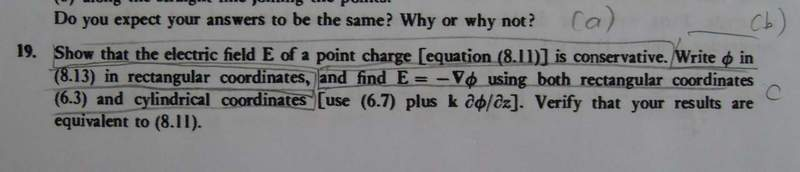## Homework Equations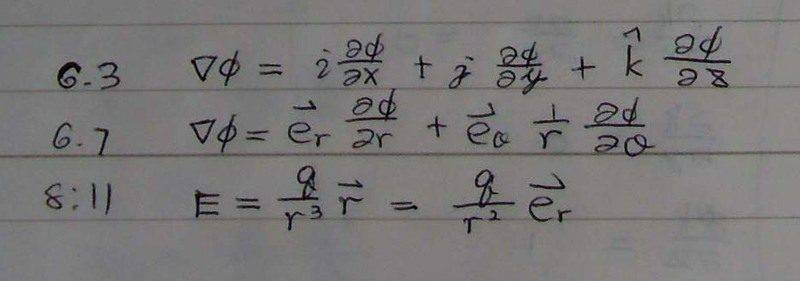## The Attempt at a Solution

I am not sure part b and c.

Also, I don't know how to answer the last question.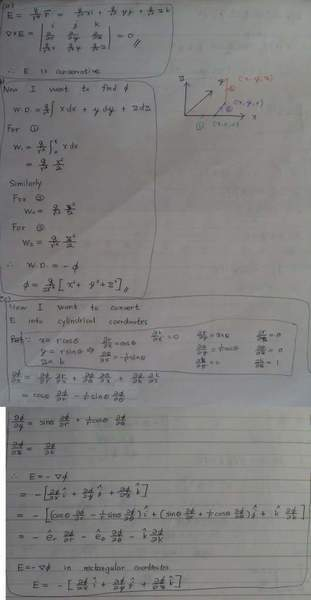#### Attachments

In your solution for part 3, you have a factor of r3 in the denominator outside the integral (in each of the three integrals). Remember r = x + y + z, so you cannot take it outside the integral.

Your approach for part c looks confusing. We'll talk about part c, let's get through part b first.

Sourabh N said:
In your solution for part 3, you have a factor of r3 in the denominator outside the integral (in each of the three integrals). Remember r = x + y + z, so you cannot take it outside the integral.

Your approach for part c looks confusing. We'll talk about part c, let's get through part b first.

It seems $r=\sqrt{x^{2}+y^{2}+z^{2}}$, isn't it?

Well if I intergrate r at the same time, it becomes $\int\frac{x}{({x^{2}+y^{2}+z^{2}})^\frac{3}{2}}$

Which is difficult to integrate...

athrun200 said:
Well if I intergrate r at the same time, it becomes $\int\frac{x}{({x^{2}+y^{2}+z^{2}})^\frac{3}{2}}$

Which is difficult to integrate...
Not really. For example, for x integral take x2 + y2 + z2 = t, then 2xdx = dt and your integral is simply dt/2*(t^1.5)

Sourabh N said:
Not really. For example, for x integral take x2 + y2 + z2 = t, then 2xdx = dt and your integral is simply dt/2*(t^1.5)

Oh. I forget that I can use substitution.
But how about the new interval?
From 0 to x^2+y^2+z^2?

athrun200 said:
Oh. I forget that I can use substitution.
But how about the new interval?
From 0 to x^2+y^2+z^2?
Common convention is to express potential relative to potential at infinity, so limits would be$\infty$ to x2 + y2 + z2.

athrun200 said:
Now, I find that the integral for x, y and z are the same.
Is that correct now?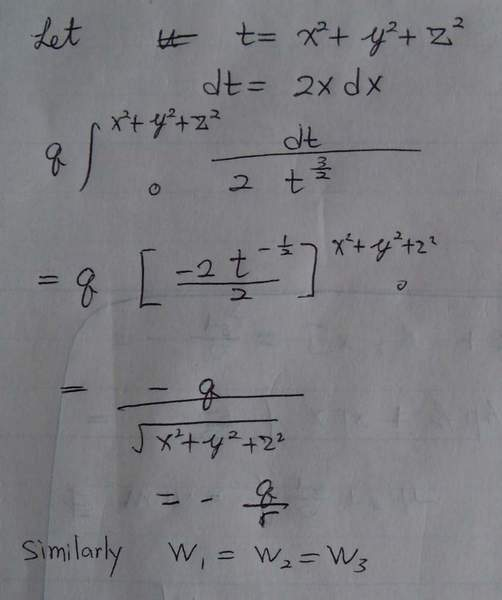2 things. First, although you wrote 0 as lower limit, you realize it's actually infinity (as I mentioned in previous post). Having the correct upper and lower limit will help you fix the sign of final value for x integral you get.
Second, note it's the x integral; it is accompanied with a $\hat{x}$ and similarly y and z integral are accompanied with respective unit vectors. Together they form $\vec{E}$ which has to look same as 8.11 (this is part c!)

Sourabh N said:
2 things. First, although you wrote 0 as lower limit, you realize it's actually infinity (as I mentioned in previous post). Having the correct upper and lower limit will help you fix the sign of final value for x integral you get.
Do you mean I should put infinty as the upper limit and x^+y^+z^2 as lower limit?
What is the physical meaning if I put infinty as the lower limit and x^+y^+z^2 as the upper limit?

Sourabh N said:
Second, note it's the x integral; it is accompanied with a $\hat{x}$ and similarly y and z integral are accompanied with respective unit vectors. Together they form $\vec{E}$ which has to look same as 8.11 (this is part c!)

I don't understand this point.
Do you mean that I miss out the vector?

However, inside the integral is a dot product. i.e.$F\bullet dr$
Since dot product produce scalar only, there should be not any vector left.

athrun200 said:
Do you mean I should put infinty as the upper limit and x^+y^+z^2 as lower limit?
What is the physical meaning if I put infinty as the lower limit and x^+y^+z^2 as the upper limit?
Look at it this way - x2 + y2 + z2 = t (where y and z and not constant anymore). So, 2xdx + 2ydy + 2zdz = dt. This substitution makes more sense than taking x2 + y2 + z2 = t with y and z constant as I had suggested previously. Because now the limit of integral is infinity and x2 + y2 + z2. I am not sure about limits of the integral if y and z are kept constant.

On the meaning of upper lower limits - Griffith defines potential like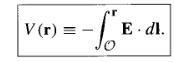I cannot possibly give a better explanation. I highly recommend you have a look (Sec 2.3 in Griffith - Intro to electrodynamics)

I don't understand this point.
Do you mean that I miss out the vector?

However, inside the integral is a dot product. i.e.$F\bullet dr$
Since dot product produce scalar only, there should be not any vector left.

Ignore this. I jumped on part c in my head.

Last edited:
Sourabh N said:
Look at it this way - x2 + y2 + z2 = t (where y and z and not constant anymore). So, 2xdx + 2ydy + 2zdz = dt. This substitution makes more sense than taking x2 + y2 + z2 = t with y and z constant as I had suggested previously. Because now the limit of integral is infinity and x2 + y2 + z2. I am not sure about limits of the integral if y and z are kept constant.

On the meaning of upper lower limits - Griffith defines potential like View attachment 37100 I cannot possibly give a better explanation. I highly recommend you have a look (Sec 2.3 in Griffith - Intro to electrodynamics)

Ignore this. I jumped on part c in my head.

So $\phi=\frac{q}{r}$?
If it is correct, let's move to part c.

I would like to know if I get the correct cylindrical form.

athrun200 said:
So $\phi=\frac{q}{r}$?
If it is correct, let's move to part c.
Yes that is correct. You can check that by using $\vec{E}$ = -$\nabla$ $\phi$ and comparing it with 8.11
I would like to know if I get the correct cylindrical form.
To get $\phi$ in cylindrical form, you have to write $\vec{E}$ in cylindrical coordinates and follow the procedure as above. (To know how to go to cylindrical from spherical, look at http://is.gd/JFitBh)

Sourabh N said:
To get $\phi$ in cylindrical form, you have to write $\vec{E}$ in cylindrical coordinates and follow the procedure as above. (To know how to go to cylindrical from spherical, look at http://is.gd/JFitBh)

Do you mean that my approach above can't get the answer?
If so, why?